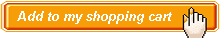Engineering System Dynamics Authors:   R. V. Dukkipati ISBN: 978-81-7319-556-3 Publication Year:   Reprint 2009 Pages:   834 Binding:   Hard Back About the book Presents in a concise but through manner fundamental statement of the theory, principles and methods for the modeling and analysis of dynamic systems. Includes concepts and review of analytical dynamics, the basic single, and two degree of freedom systems using the energy and matrix methods, review of classical matrix analysis, Laplace transforms, modeling of dynamic systems, the performance and stability, and frequency response methods for the analysis and design of feedback control systems. Key Features Systematic approach for the analysis of dynamic systems • Illustrative examples • Programming examples using MATLAB software Table of content Preface / Introduction /Matrix Analysis/Laplace Transforms/Mathematical Modeling of Dynamic Systems/Single Degree of Freedom Systems/Two Degree of Freedom Systems/ Introduction to Control Systems/System Representation/Performance and Stability of Feedback Systems/Analysis of Control Systems/Design of Control Systems/ MATLAB Basics/ MATLAB Tutorial / Appendix A: Complex Analysis / Appendix B: Vector Algebra / Appendix C: Units and Conversion / Glossary of Terms / Glossary of Symbols / Answers to Selected Problems / Bibliography / IndexAudience Undergraduate and Postgraduate Students and Practicing Engineers